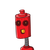# The common difference of an ap is 5/2. What would be the difference of its 15th term and 5th term ?

The common difference of an ap is 5/2. What would be the difference of its 15th term and 5th term ?

### 1 thought on “The common difference of an ap is 5/2. What would be the difference of its 15th term and 5th term ?”

1.## Step-by-step explanation:

AP = a1 , a2 , a3 , a4

AP = a , a+d , a+2d , a+3d

d = 5/2

15th term – a15 = a + 14d

5th term  –  a5 = a + 4d

a15 – a14

= a + 14d – (a + 4d)

= a + 14d – a 4d

= 14d – 4d

= 10d

= 10 × 5 / 2

= 5 × 5

= 25